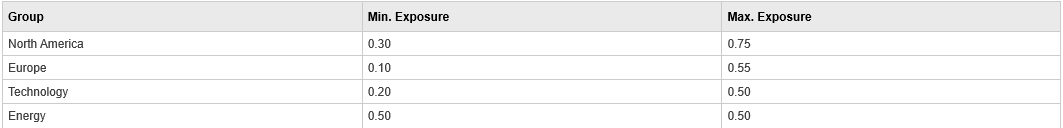# pcglims

Linear inequalities for asset group minimum and maximum allocation

## Syntax

``[A,b] = pcglims(Groups,GroupMin,GroupMax)``

## Description

As an alternative to `pcglims`, use the Portfolio object (`Portfolio`) for mean-variance portfolio optimization. This object supports gross or net portfolio returns as the return proxy, the variance of portfolio returns as the risk proxy, and a portfolio set that is any combination of the specified constraints to form a portfolio set. For information on the workflow when using Portfolio objects, see Portfolio Object Workflow.

example

````[A,b] = pcglims(Groups,GroupMin,GroupMax)` specifies minimum and maximum allocations to groups of assets. Bounds can be specified for an arbitrary number of groups `NGROUPS`,made up as subsets of the `NASSETS` investments.If `pcglims` is called with fewer than two output arguments, the function returns `A` concatenated with `b` `[A,b]`.```

## Examples

collapse all

Use the following assets and groupings.Set the minimum and maximum investment in various groups.

```% INTC XOM RD Groups = [ 1 1 0 ; % North America 0 0 1 ; % Europe 1 0 0 ; % Technology 0 1 1 ]; % Energy GroupMin = [0.30 0.10 0.20 0.50]; GroupMax = [0.75 0.55 0.50 0.50]; [A,b] = pcglims(Groups, GroupMin, GroupMax)```
```A = 8×3 -1 -1 0 0 0 -1 -1 0 0 0 -1 -1 1 1 0 0 0 1 1 0 0 0 1 1 ```
```b = 8×1 -0.3000 -0.1000 -0.2000 -0.5000 0.7500 0.5500 0.5000 0.5000 ```

Portfolio weights of 50% in INTC, 25% in XOM, and 25% in RD satisfy the constraints.

## Input Arguments

collapse all

Grouping, specified as a number of groups (`NGROUPS`) by number of assets (`NASSETS`) vector of which assets belong to which group. Each row specifies a group. For a specific group, `Group(i,j) = 1` if the group contains asset `j`; otherwise, `Group(i,j) = 0`.

Data Types: `double`

Minimum allocations, specified as a scalar or `NGROUPS`-long vectors of minimum combined allocations in each group. `NaN` indicates no constraint. Scalar bounds are applied to all groups.

Data Types: `double`

Maximum allocations, specified as a scalar or `NGROUPS`-long vectors maximum combined allocations in each group. `NaN` indicates no constraint. Scalar bounds are applied to all groups.

Data Types: `double`

## Output Arguments

collapse all

Lower bound, returned as a matrix such that ```A*PortWts' <= b```, where `PortWts` is a `1`-by-`NASSETS` vector of asset allocations.

Upper bound, returned as a vector such that ```A*PortWts' <= b```, where `PortWts` is a `1`-by-`NASSETS` vector of asset allocations.

Introduced before R2006a